Ex 3.3

Chapter 3 Class 7 Data Handling
Serial order wise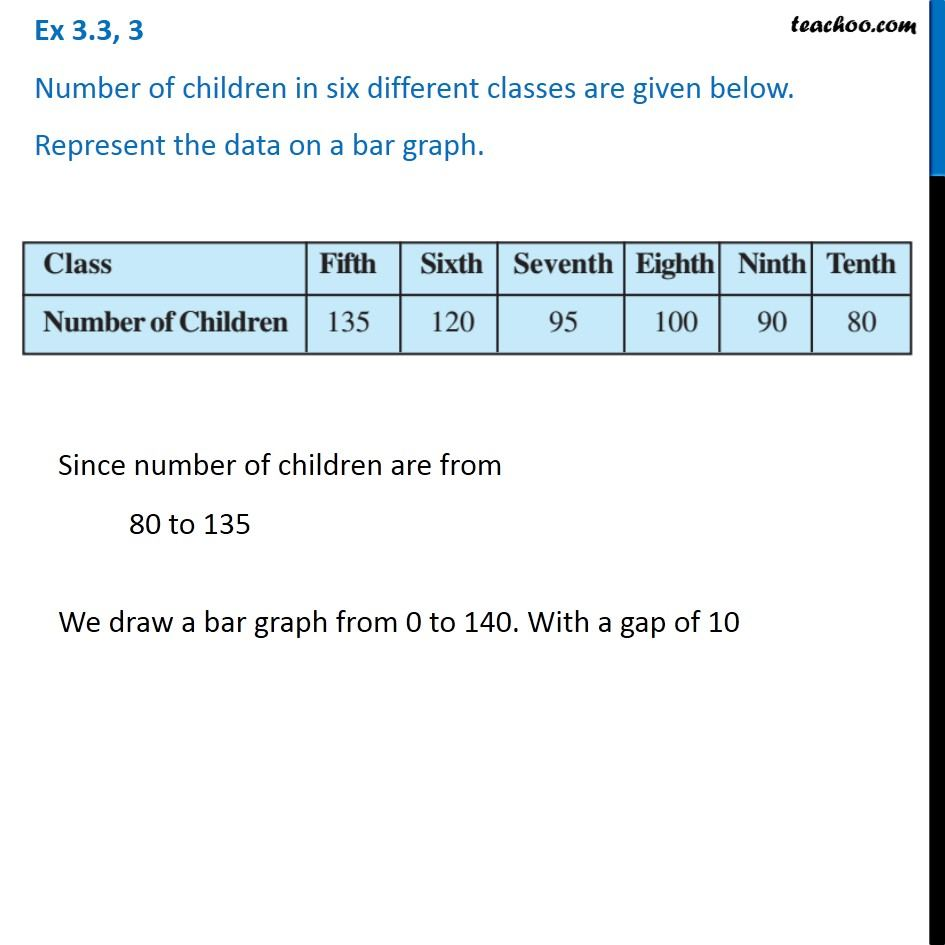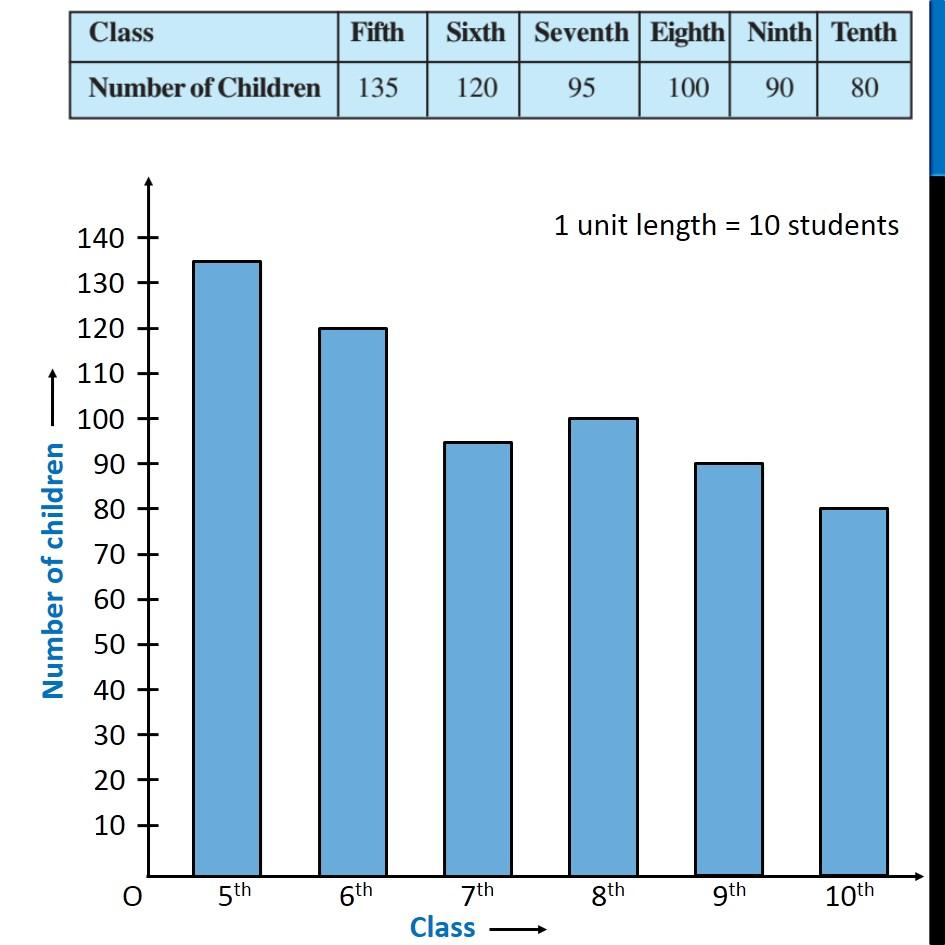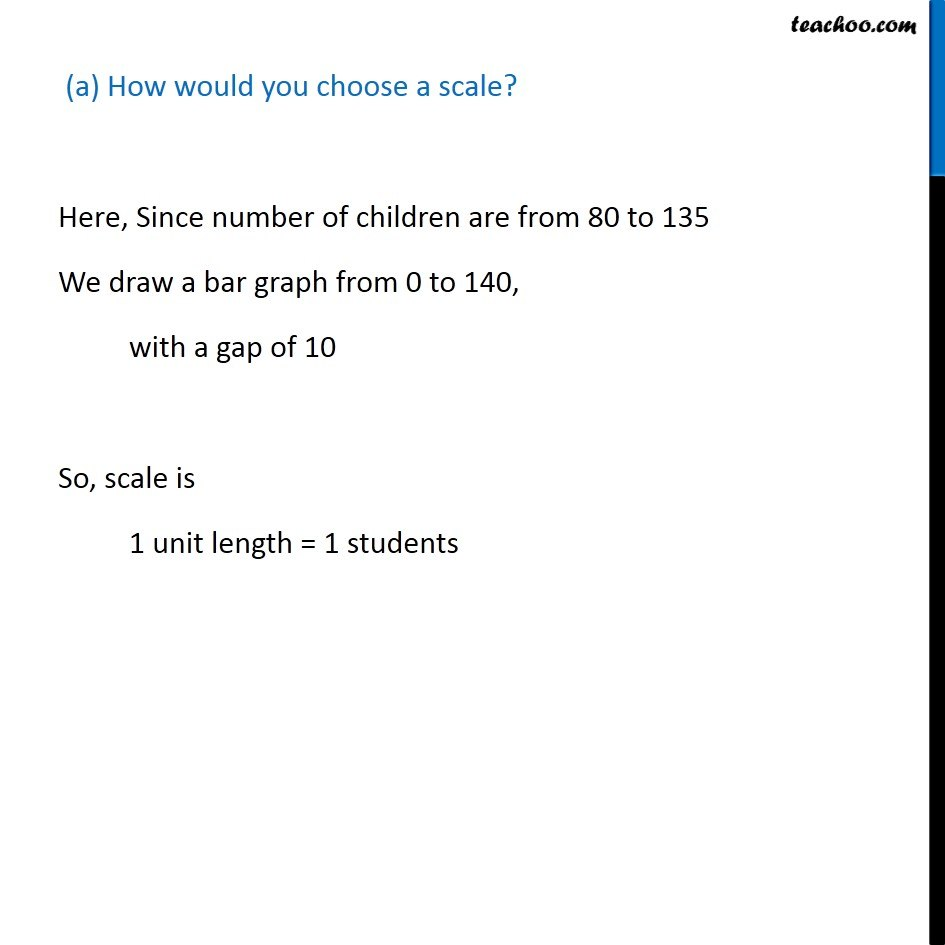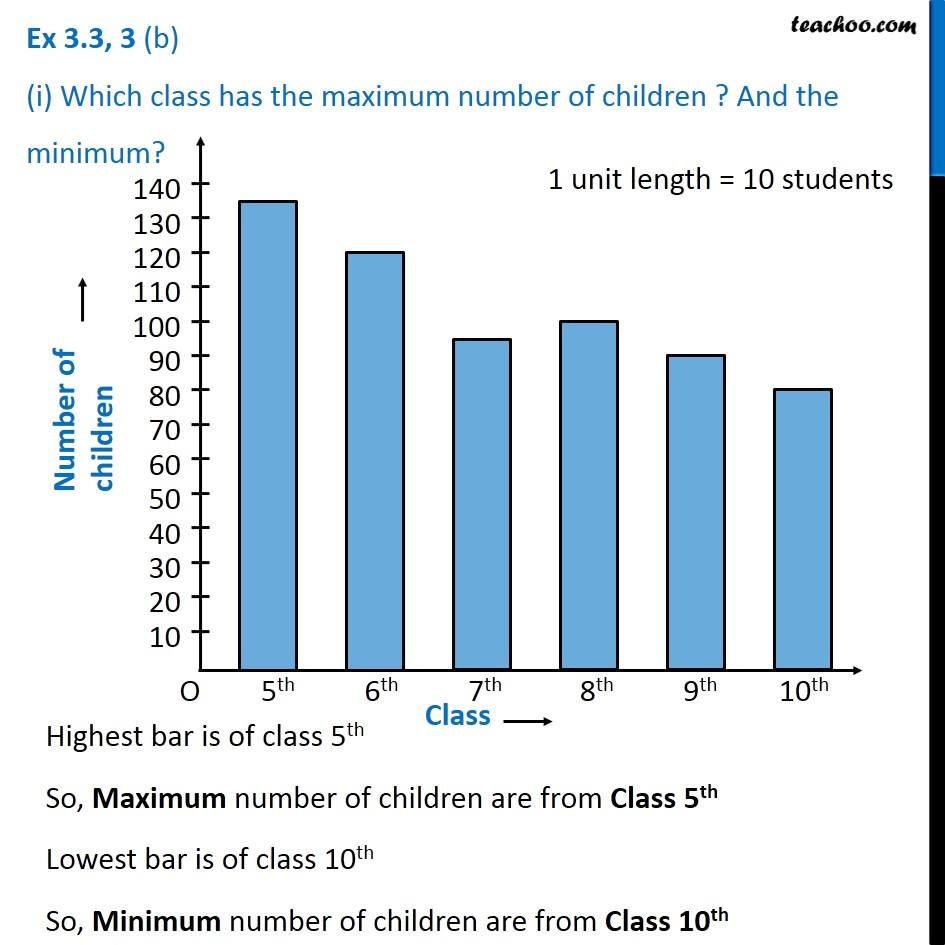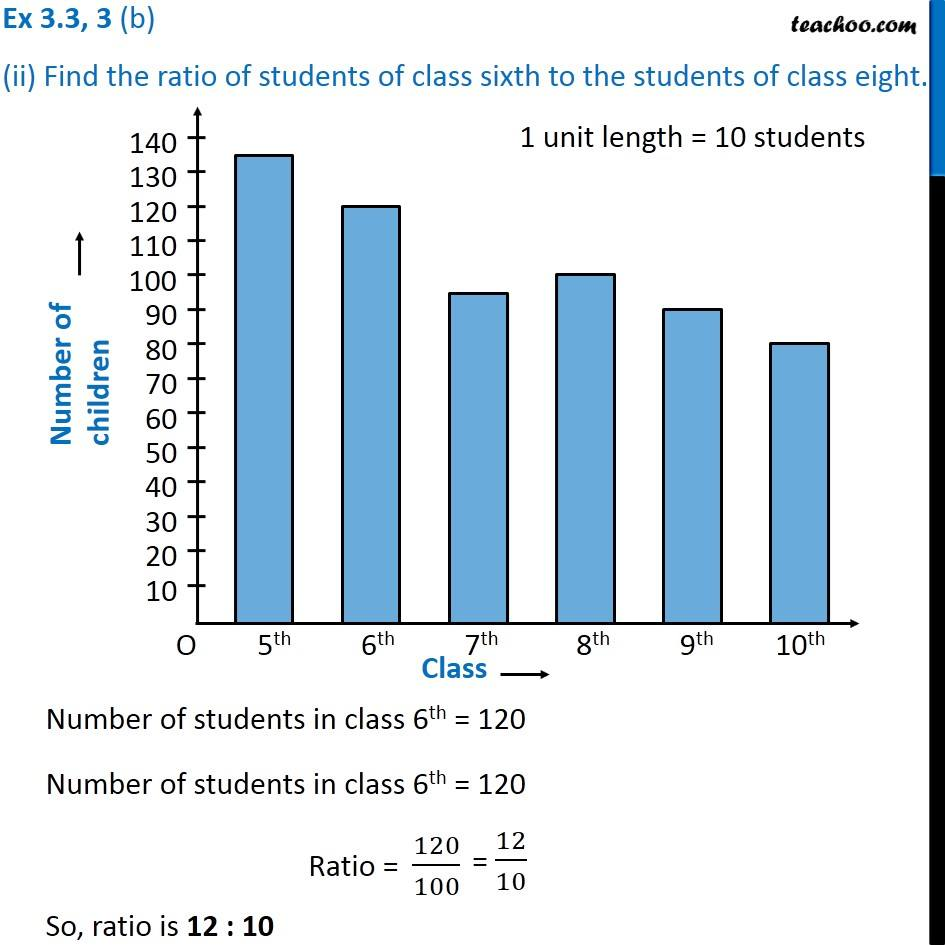Learn in your speed, with individual attention - Teachoo Maths 1-on-1 Class

### Transcript

Ex 3.3, 3 Number of children in six different classes are given below. Represent the data on a bar graph. Since number of children are from 80 to 135 We draw a bar graph from 0 to 140. With a gap of 10 (a) How would you choose a scale? Here, Since number of children are from 80 to 135 We draw a bar graph from 0 to 140, with a gap of 10 So, scale is 1 unit length = 1 students Ex 3.3, 3 (b) (i) Which class has the maximum number of children ? And the minimum Highest bar is of class 5th So, Maximum number of children are from Class 5th Lowest bar is of class 10th So, Minimum number of children are from Class 10th Ex 3.3, 3 (b) (ii) Find the ratio of students of class sixth to the students of class eight.Number of students in class 6th = 120 Number of students in class 6th = 120 Ratio = 120/100 So, ratio is 12 : 10